# Exclusive disjunction facts for kids

Kids Encyclopedia Facts
(Redirected from Exclusive or)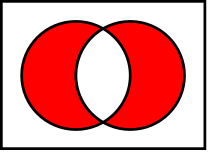Venn diagram of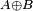$A \oplus B$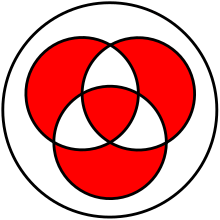Venn diagram of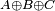$A \oplus B \oplus C$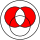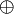$~\oplus~$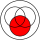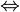$~\Leftrightarrow~$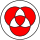Exclusive disjunction (also called exclusive or, XOR) is a Logic operation on two values. It will be true, if exactly one of the two values is true. Otherwise it will be false. This also means that the result of 'XOR' will be true only if both the values are different. Same values will result in a false.

The best way to remember a XOR operation is: "One or the other, but not both".

This is different from inclusive disjunction.

## Truth table

The truth table of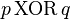$p\, \mathrm{XOR}\, q$ (also written as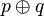$p \oplus q$, or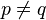$p \neq q$) is as follows:

p q
F F F
F T T
T F T
T T FExclusive disjunction Facts for Kids. Kiddle Encyclopedia.